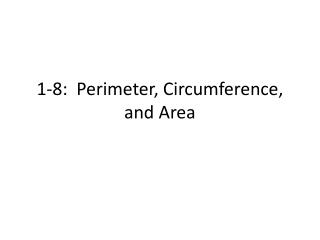# 1-8: Perimeter, Circumference, and Area - PowerPoint PPT PresentationDownload Presentation1-8: Perimeter, Circumference, and Area

1-8: Perimeter, Circumference, and Area
Download Presentation## 1-8: Perimeter, Circumference, and Area

- - - - - - - - - - - - - - - - - - - - - - - - - - - E N D - - - - - - - - - - - - - - - - - - - - - - - - - - -
##### Presentation Transcript

1. 1-8: Perimeter, Circumference, and Area

2. Perimeter and Area • The perimeterP of a polygon is the sum of the lengths of its sides. • Distance around the outside of a figure • Measured in inches, feet, yards, miles, meters, etc. • The areaA of a polygon is the number of square units it encloses. • Amount inside of a figure • Measured in square inches (in.2), square feet (ft2), square yards (yd2), square miles (mi2), square meters (m2), etc.

3. Common Formulas

4. Finding the Perimeter of a Rectangle The botany club members are designing a rectangular garden for the courtyard of the school. They plan to place edging on the outside of the path. How much edging material will they need?

5. Circles • You can name a circle with the symbol  and the capital letter that represents its center. • For example, a circle with center A is written A. • Formulas for circles involve the special number pi (). • Pi is the ratio of a circle’s circumference to its diameter and is irrational (a nonterminating decimal). • Pi can be approximated as 3.14 or 22/7, or you can use the pi button on your calculator.

6. Finding Circumference What is the circumference of the circle in terms of ? …rounded to the nearest tenth?

7. Finding the Area of a Rectangle You want to make a rectangular banner. It should be 2 ½ ft wide and 5 ft high. To the nearest square yard, how much material do you need?

8. Finding Area of a Circle What is the area of K in terms of ?

9. Finding Area of an Irregular Shape Area Addition Postulate: The area of a region is the sum of the areas of its nonoverlapping parts. • What is the area of the figure? All angles are right angles.

10. 10-3 Areas of Regular Polygons

11. Regular Polygons You can circumscribe a circle about any regular polygon. The center of a regular polygon is the center of this circle. The radius of a regular polygon is the distance from the center to a vertex. The apothem is the perpendicular distance from the center.

12. Finding Angle Measures • The figure is a regular pentagon with radii and an apothem drawn. What is the measure of each numbered angle?

13.  A portion of a regular octagon is shown with radii and an apothem drawn. What is the measure of each numbered angle?

14. Equilateral Triangles (not in book) The area of ANY triangle is given by A = ½ bh, if you are provided (or find) the lengths of the base and height. However, you can find the area of an equilateral triangle using only the side length. Area of an Equilateral Triangle The area of an equilateral triangle is one fourth the square of the length of the side times √3. s s s

15. Finding the Area of an Equilateral Triangle Find the area of an equilateral triangle with 8 inch sides.  Find the area of an equilateral triangle with 10 cm sides.

16. Area of Regular Polygons Postulate: If two figures are congruent, then their areas are equal. Area of a Regular Polygon Theorem: The area of a regular polygon is half the product of the apothem and the perimeter. A = ½aP *Since a hexagon is made up of 6 equilateral triangles, you can find the area using the equilateral triangle formula multiplied by 6.

17. Finding the Area of a Regular Polygon • What is the area of the regular decagon?

18. What is the area of a regular pentagon with an 8-cm apothem and 11.6-cm sides?

19. Finding the Area of a Regular Hexagon • The side of a regular hexagon is 3 mm. What is the area of the hexagon? The side of a regular hexagon is 16 ft. What is the area of the hexagon?